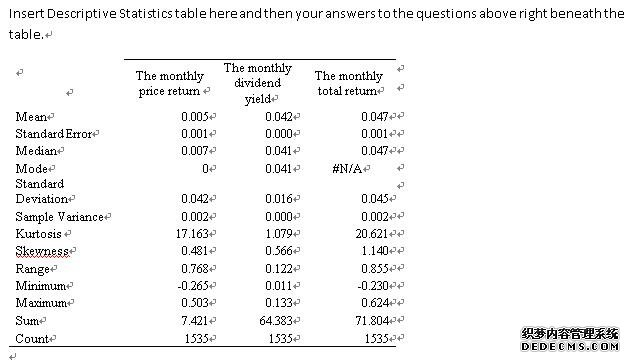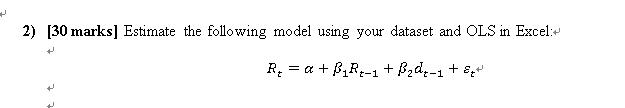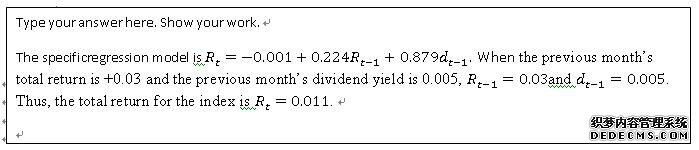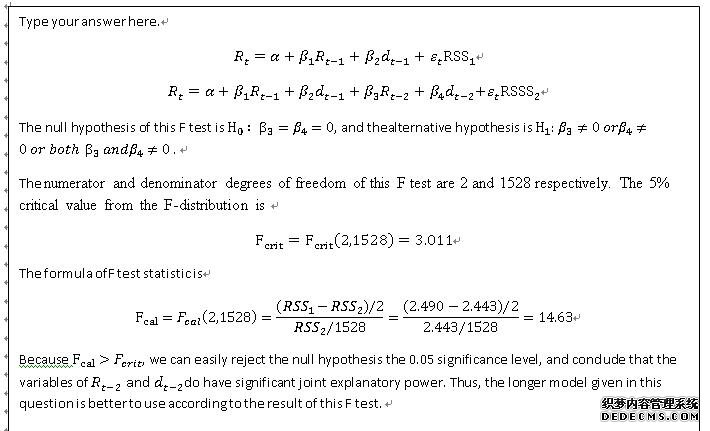# 英国Fiance Coursework：explore whether past dividend yields and

 在本课程中，我们的目标是探讨过去的股息收益率和股票回报率是否能预测股票回报率。数据集都在讨论中。   1）下载与您的作业对应的Excel文件。该文件名为“Shiller Data–CourseWork DataSet.xlsx”。 2）请您回答以下四个问题。填写这份Word文档并将其作为你的课程作业提交。保留本文档的页边空白和每个问题下面给出的模板，并使用11磅。在写答案时字体或更大。请记住，字迹不清或格式不正确的答案将要付出代价。 [20分]在您的Excel文件中，B列包含股票市场指数的月末价值，C列包含指数中所有股票的每月总股息支付，均以名义美元支付。开始月份不同于数据集，但结束月份在所有情况下都是2016年12月。In this coursework, our goal is to explore whether past dividend yields and past stock returns can predict stock returns. The data sets are all on Moodle, under Week 19. 1) Download the Excel file that corresponds to your assignment. The file has the name “Shiller data – coursework dataset.xlsx”.  2) You are asked to answer the four questions below. Fill in this Word document and submit it as your coursework. Keep the margins of this documentand the templates given underneath each question as they are and use a 11 pt. font or larger when writing up your answers. Keep in mind that illegible or badly formatted answers will cost marks.   COURSEWORK QUESTIONS课程作业问题 [20分]在您的Excel文件中，B列包含股票市场指数的月末价值，C列包含指数中所有股票的每月总股息支付，均以名义美元支付。开始月份不同于数据集，但结束月份在所有情况下都是2016年12月。 [20 marks]In your Excel file,column B contains the end-month value of a stock market index and column C contains the monthly aggregate dividend payment from all stocks in the index, both in nominal dollars. The starting month differs from data set to data set, but the ending month is December 2016 in all cases.确定整个时间段的rt、dt和rt，并在下框中的表格中生成其样本统计数据（平均值、中位数、标准偏差、偏度、峰度）。然后，在同一个框中再次回答以下问题： 哪一个系列更具变化性，价格回报率还是股息收益率？你对价格回报率序列的偏态统计有何解释？在这一时期投资股市指数是一个好的投资决策吗？证明你的回答是正确的。Determine rt, dt and Rtfor the entire time period and produce their sample statistics (mean, median, standard deviation, skewness, kurtosis) in a table in the box below. Then, answer the following questions, again in the same box:  Which series is more variable, the price return or the dividend yield? What is your interpretation of the skewness statistics for the price return series? Does investing in the stock market index in this period appear to be a good investment decision? Justify your answer.The standard deviation andvariance can describe the volatility of variable. The greater value of the variance, the higher volatility of the series. The standard deviation of the price return and the dividend yield are 0.042 and 0.016 respectively. The price return has the higher standard deviation and volatility. Thus, the series of the monthly price return is more variable. The skewness can measure the asymmetry of the probability distribution of series about mean value.  Ifskewness>0, there is positive skew. If skewness<0, there is negative skew. If the skewness close to zero, the distribution is symmetry. The skewness of price return is 0.481>0, the series of price return is positive skew.  The mean value of the price return and the dividend yield are 0.005 and 0.0042, that is, the monthly total returnis  0.047. In addition, the standard deviation of the monthly total return is 0.045, which is relative smaller. Thus, the stock market index in this period appear to be gain profit but with low risks. Therefore, investing in the stock market index in this period appear to be a good investment decision.where  ε_tis an error term. Note that your independent variables are the total return and dividend yield from the previous month. Copy and paste your Excel regression output in the box provided below:Is the model able to explain index returns with some success? Comment using the r-square from the OLS output. Comment on this outcome in the context of market efficiency, i.e. would you expect stock returns to be predictable when one uses past data that is easily obtainable?    Type your answer here. The r-square from the regression model is 0.205, which is relatively low. Thus, the good of fitness is relative low. In other words, only 20.5% of the variation in our dependent variable is explained by the model. The remaining 79.5% is due to noise, i.e. the cumulative effect of factors we have not included in the model. Thus, the model is not able to explain index return with some success. Even though the good of fitness is relatively low, this model has two individually statistically insignificant coefficient estimates. Thus, the model has relatively market efficiency.    Predict the total return for the index when the previous month’s total return is +0.03 and the previous month’s dividend yield is 0.005.3) [15 marks]Test thenull hypothesis that β_2=1using a two-tailed test. Show your calculation of the test statistic. Make sure that you state the null and alternative hypotheses, the degrees of freedom used and the critical value from the Student’s t-distribution.4) [35 marks] Wewill now examine if adding one more lag of returns and dividend yields to the model above will improve its explanatory power. Run the following model in Excel:Perform an F-test to see if we should pick the shorter model from Q2 or the longer model given in this question. Carefully state your null and alternative hypotheses, the numerator and denominator degrees of freedom you are using to determine the critical value from the F-table, and the 5% critical value from the F-distribution. Which model is better to use according to the result of your F-test?How could we proceed, along the lines of the modification to the model in Q2 that we made above in this question, in our search for better explanatory power? Briefly explain.    Type your answer here. The time series of the monthly dividend yield and the monthly total return maybe not stationary. In order to process in our search, we can translate the time series of both the monthly dividend yield and the monthly total return into logarithmic form, and then make regression model using the logarithmic form.